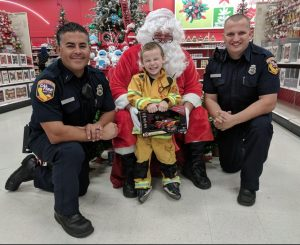[vfb id=3]

## Family Night Out Event

this is sample content this is sample content this is sample content this is sample content this is sample content this is sample content this is sample content this is sample content this is sample content this is sample content this is sample content this is sample content this is sample content this is sample content this is sample content this is sample content this is sample content this is sample content this is sample content this is sample content this is sample content this is sample content this is sample content this is sample content this is sample content this is sample content

this is sample content this is sample content this is sample content this is sample content this is sample content this is sample content this is sample content this is sample content this is sample content this is sample content this is sample content this is sample content this is sample content this is sample content this is sample content this is sample content this is sample content this is sample content this is sample content this is sample content this is sample content this is sample content this is sample content this is sample content this is sample content this is sample content this is sample content this is sample content this is sample content this is sample content this is sample content this is sample content this is sample content this is sample content this is sample content this is sample content this is sample content this is sample content this is sample content this is sample content this is sample content this is sample content this is sample content this is sample content this is sample content this is sample content this is sample content

## Shop With A Fire Fighterthis is sample content this is sample content this is sample content this is sample content this is sample content this is sample content this is sample content this is sample content this is sample content this is sample content this is sample content this is sample content this is sample content this is sample content this is sample content this is sample content this is sample content this is sample content this is sample content this is sample content this is sample content this is sample content this is sample content this is sample content this is sample content this is sample content this is sample content this is sample content this is sample content this is sample content this is sample content this is sample content this is sample content this is sample content this is sample content this is sample content

this is sample content this is sample content this is sample content this is sample content this is sample content this is sample content this is sample content this is sample content this is sample

Shop With A Fire Fighter Request Application

[vfb id=1]